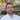# Chialisp Basics

May 24, 2021

Chialisp is the native smart contract language of the Chia blockchain and cryptocurrency (XCH). This post demonstrates basic Chialisp commands, operators, and an example program. It is based on this official video: An Introduction to developing in Chialisp.

I’ve included a few updates/corrections based on things that have changed. For example, the quoting syntax was changed from this `brun '(+ (q 2) (q 3))'` to this `brun '(+ (q . 2) (q . 3))'`

## Setup

Follow steps here: https://github.com/Chia-Network/clvm_tools

## Commands

``````brun '(q 100)'
(100)

brun '(+ (q . 2) (q . 3))'
5

run '(+ 2 3)'
5

brun '(* (q . 3) (q . 4) (q . 5))'
60

brun '(i (q . 1) (q . "true") (q . "false"))'
"true"

brun '(i (q . 0) (q . "true") (q . "false"))'
"false"

brun '(i (q . ()) (q . "true") (q . "false"))'
"false"

brun '(sha256 (q . "asdf"))'
0xf0e4c2f76c58916ec258f246851bea091d14d4247a2fc3e18694461b1816e13b

brun '(x)'
FAIL: clvm raise ()

# `1` references the input argument list
brun '1' '(200 300 400 "hey")'
(200 300 400 "hey")

# `2` references the first element in input argument list
brun '2' '(200 300 400 "hey")'
200

brun '(f 1)' '(1 2 3 "a")'
1

brun '(i (= (f 1) (q . 100)) (q . "true") (q . "false"))' '(100)'
"true"

brun '(i (= (f 1) (q . 100)) (q . "true") (q . "false"))' '(200)'
"false"``````

## Basic Program

This is the same example from the official docs: Password Locked Coin

Get the hash of “hello” which will be the password required to spend the coin:

``````brun '(sha256 (q . "hello"))'
0x2cf24dba5fb0a30e26e83b2ac5b9e29e1b161e5c1fa7425e73043362938b9824``````

Here’s the program:

``````; demo.clvm
(mod (password puzzhash amount)
(if (= (sha256 password) (q . 0x2cf24dba5fb0a30e26e83b2ac5b9e29e1b161e5c1fa7425e73043362938b9824))
(list (list 51 puzzhash amount))
(x "wrong password")
)
)``````

Compile the program:

``````run demo.clvm
(a (i (= (sha256 2) (q . 0x2cf24dba5fb0a30e26e83b2ac5b9e29e1b161e5c1fa7425e73043362938b9824)) (q 4 (c (q . 51) (c 5 (c 11 ()))) ()) (q 8 (q . "wrong password"))) 1)``````

We can copy and paste the output from the command above and use it as the first argument to `brun`, or use command substitution (with double quotes) which is much easier to reason about.

Test with the correct password “hello”. It should succeed and output the list of conditions.

``````brun "\$(run demo.clvm)" '("hello" 0xcafef00d 200)'
((51 0xcafef00d 200))``````

Test with an incorrect password. It should raise an error.

``````brun "\$(run demo.clvm)" '("helloops" 0xcafef00d 200)'
FAIL: clvm raise ("wrong password")``````

## Referencing Input Arguments

Input arguments, or `treeargs` in Chialisp parlance.

How to reference `treeargs` is explained really well here: Treeargs : Program Arguments, and Argument Lookup

## Questions / TODO

### 5

In the official docs, Password Locked Coin, it mentions the following:

`5` is equivalent to `(f (r 1))`

So `5` takes the tail of the input list, and then takes the head of that. In other words, `5` takes the second element from the input list.

TODO: Why? Where is this in the compiler?

Here’s an example of using `5`:

``````brun '5' '(101 102 103 104 105)'
102``````

### qq and unquote

`chia/wallet/puzzles/p2_conditions.clvm`

``````(mod (conditions)
(qq (q . (unquote conditions)))
)``````

### l

Returns true if the argument is a cons box (or list). Returns false if the argument is an atom.

``````run '(l (f @))' '("atom" ("a" "list"))'
()

run '(l (r @))' '("atom" ("a" "list"))'
1

brun '(l 2)' '("atom" ("a" "list"))'
()

brun '(l 3)' '("atom" ("a" "list"))'
1``````

## References

• CLVM Reference Manual - Official CLVM documentation, in depth explanations of the inner workings of Chialsip and the CLVMSoftware engineer crafting full-stack, cloud-native solutions for enterprise. GitHub | LinkedIn | Twitter